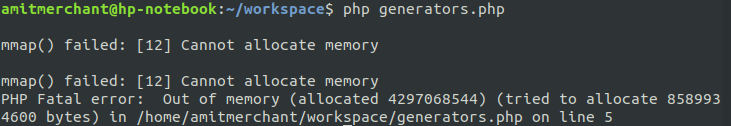# Amit MerchantVerified (\$10/year for the domain)

A blog on PHP, JavaScript, and more

# A deep dive into Generators in PHP

July 31, 2019 ·

Have you ever stuck in a situation where the code that you’ve wrote uses foreach to iterate over a set of data into an array and which ultimately caused you to exceed a memory? Well, I’ve been to. Many times.

Take this for example:

``````<?php
function generateNumbers(\$number) {
\$build = [];
for (\$i = 1; \$i <= \$number; \$i++) {
\$build[] = \$i;
}
return \$build;
}

\$result = generateNumbers(5);
foreach (\$result as \$value) {
echo "Number: \$value\n";
}
``````

As you can see, we’ve a function `generateNumbers` which basically accepts a number as its only parameter and will build an array which stores the range of numbers and returns the array ultimately and then we can loop over the result of that function. In our example, it will generate an array which contains range of number from 1 to 5.This will work fine for the small amount of `\$number`. But what if we want to generate a really big range of numbers? Let’s say `generateNumbers(INF)`. Running the above script by replacing the number with `INF` (This is a predefined constant which represents infinity in PHP). Let’s see what happens.As you can see, PHP has thrown “Out of memory” fatal error. Obvious solution here would be to increase `memory_limit` by going into php.ini. But PHP has better solution for this situation. Enter “Generators”.

## What are generators?

Generators are like the normal functions in PHP but instead of returning a value, they yields as many values as it needs to. So, whichever function that contains “yield” is a generator.

When a generator function is called, it returns an object that can be iterated over. Let’s understand this by modifying the earlier example by using generator.

``````<?php
function generateNumbers(\$number) {
for (\$i = 1; \$i <= \$number; \$i++) {
yield \$i;
}
}

foreach (generateNumbers(100000000000) as \$value) {
echo "Number: \$value\n";
}
``````

As you can see here, the function `generateNumbers` is become a generator and now it can be iterated directly into the `foreach` loop. The `generateNumbers` function will return a `Generator` object which is iterable using regular loops. When you iterate over that object (for instance, via a foreach loop), PHP will call the object’s iteration methods each time it needs a value, then saves the state of the generator when the generator yields a value so that it can be resumed when the next value is required.

It’s like returning the value from a function in “realtime” and you don’t need to maintain the state of the values in the function itself. And once there are no more values to be yielded, then the generator can simply exit, and the calling code continues just as if an array has run out of values.

## Role of the `yield` keyword

The meaning of “yielding” means “To produce or provide”. It works exactly the same way in terms of generators as its meaning. In generators, a yield statement looks much like a return statement, except that instead of stopping execution of the function and returning, yield instead provides a value to the code looping over the generator and pauses execution of the generator function.

Apart from yielding only values from generators, you’d also can return key/value pair from the generators. Here’s how it looks for the earlier example.

``````<?php
function generateNumbers(\$number) {
for (\$i = 0; \$i <= \$number; \$i++) {
yield \$i => 'value-'.\$i;
}
}

foreach (generateNumbers(5) as \$key => \$value) {
echo "Number\$key: \$value\n";
}
``````

## Yielding null values

Yield can be called without an argument to yield a NULL value with an automatic key.

``````<?php
function gen_three_nulls() {
foreach (range(1, 3) as \$i) {
yield;
}
}

var_dump(iterator_to_array(gen_three_nulls()));
``````

This will generate array of `null` values like this

``````array(3) {
=>
NULL
=>
NULL
=>
NULL
}
``````

Another interesting point that can be noted here is, you can `return` a value from a generator at the same time. The returned value can be retrieved using `getReturn()` method of `Generator` object.

``````function fetchData()
{
foreach (range(1, 3) as \$i) {
yield \$i;
}
return true;
}

foreach(fetchData() as \$value) {
echo \$value;
}

print_r(fetchData()->getReturn()); // This will print "true"
``````

## Conclusion

Generators are great when you’re working with the large set of data which can eat up your memory if utilized using some other means such as normal function which return values. If used wisely, Generator can make those memory eating scripts to bare minimum and in turn give your application a certain amount of performance boost.

👋 Hi there! I'm Amit. I write articles about all things web development. If you like what I write and want me to continue doing the same, I would like you buy me some coffees. I'd highly appreciate that. Cheers!My Tiny YouTube Channel ⟶